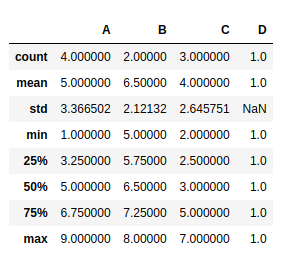# How to count the number of NaN values in Pandas?

We might need to count the number of NaN values for each feature in the dataset so that we can decide how to deal with it. For example, if the number of missing values is quite low, then we may choose to drop those observations; or there might be a column where a lot of entries are missing, so we can decide whether to include that variable at all.

Method 1: Using describe()

We can use the describe() method which returns a table containing details about the dataset. The count property directly gives the count of non-NaN values in each column. So, we can get the count of NaN values, if we know the total number of observations.

 `import` `pandas as pd  ` `import` `numpy as np ` `   `  `# dictionary of lists  ` `dict` `=` `{ ``'A'``:[``1``, ``4``, ``6``, ``9``],  ` `        ``'B'``:[np.NaN, ``5``, ``8``, np.NaN],  ` `        ``'C'``:[``7``, ``3``, np.NaN, ``2``], ` `        ``'D'``:[``1``, np.NaN, np.NaN, np.NaN] }  ` ` `  `# creating dataframe from the ` `# dictionary  ` `data ``=` `pd.DataFrame(``dict``)  ` `   `  `data.describe()`

Output :Method 2: Using sum()
The isnull() function returns a dataset containing True and False values. Since, True is treated as a 1 and False as 0, calling the sum() method on the isnull() series returns the count of True values which actually corresponds to the number of NaN values.

Counting NaN in a column :

We can simply find the null values in the desired column, then get the sum.

 `import` `pandas as pd ` `import` `numpy as np ` `   `  `# dictionary of lists  ` `dict` `=` `{ ``'A'``:[``1``, ``4``, ``6``, ``9``],  ` `        ``'B'``:[np.NaN, ``5``, ``8``, np.NaN],  ` `        ``'C'``:[``7``, ``3``, np.NaN, ``2``], ` `        ``'D'``:[``1``, np.NaN, np.NaN, np.NaN] }  ` `   `  `# creating dataframe from the ` `# dictionary  ` `data ``=` `pd.DataFrame(``dict``)  ` ` `  `# total NaN values in column 'B' ` `print``(data[``'B'``].isnull().``sum``())`

Output :

`2`

Counting NaN in a row :

The row can be selected using loc or iloc. Then we find the sum as before.

 `import` `pandas as pd  ` `import` `numpy as np ` `   `  `# dictionary of lists  ` `dict` `=` `{ ``'A'``:[``1``, ``4``, ``6``, ``9``], ` `        ``'B'``:[np.NaN, ``5``, ``8``, np.NaN],  ` `        ``'C'``:[``7``, ``3``, np.NaN, ``2``], ` `        ``'D'``:[``1``, np.NaN, np.NaN, np.NaN] }    ` `   `  `# creating dataframe from the  ` `# dictionary  ` `data ``=` `pd.DataFrame(``dict``)  ` ` `  `# total NaN values in row index 1 ` `print``(data.loc[``1``, :].isnull().``sum``())`

Output :

`1`

Counting NaN in the entire DataFrame :
To count NaN in the entire dataset, we just need to call the sum() function twice – once for getting the count in each column and again for finding the total sum of all the columns.

 `import` `pandas as pd  ` `import` `numpy as np ` `   `  `# dictionary of lists  ` `dict` `=` `{``'A'``:[``1``, ``4``, ``6``, ``9``], ` `        ``'B'``:[np.NaN, ``5``, ``8``, np.NaN], ` `        ``'C'``:[``7``, ``3``, np.NaN, ``2``], ` `        ``'D'``:[``1``, np.NaN, np.NaN, np.NaN]}  ` `   `  `# creating dataframe from the ` `# dictionary  ` `data ``=` `pd.DataFrame(``dict``)  ` ` `  `# total count of NaN values ` `print``(data.isnull().``sum``().``sum``())`

Output :

`6`

Attention geek! Strengthen your foundations with the Python Programming Foundation Course and learn the basics.

To begin with, your interview preparations Enhance your Data Structures concepts with the Python DS Course.

My Personal Notes arrow_drop_upCheck out this Author's contributed articles.

If you like GeeksforGeeks and would like to contribute, you can also write an article using contribute.geeksforgeeks.org or mail your article to contribute@geeksforgeeks.org. See your article appearing on the GeeksforGeeks main page and help other Geeks.

Please Improve this article if you find anything incorrect by clicking on the "Improve Article" button below.

Article Tags :

Be the First to upvote.

Please write to us at contribute@geeksforgeeks.org to report any issue with the above content.